# Draw the shear force and bending moment diagrams for beams

• DevonZA
In summary, the homework equations state that downward forces are negative, while upward forces are positive. Moments about points are calculated as force multiplied by perpendicular distance. Anti clockwise moments are positive.f

## Homework Statement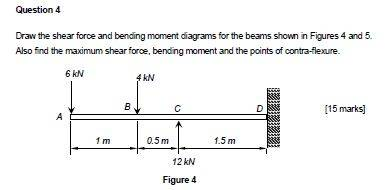## Homework Equations

[/B]
Shear force is calculated at each point on the beam. Downward forces are negative, upward forces are positive.

Moments about points are calculated as force multiplied by perpendicular distance. Clockwise moments are negative. Anti clockwise moments are positive.

## The Attempt at a Solution

SF @ A = -6kN
SF @ B = -4kN
SF @ C = 12kN
SF @ D = 12kN (same as C)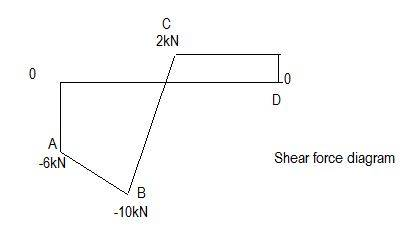BM@A=0
BM@B=6kNx1+12kNx0.5 = 12kN CCW
BM@C=6kNx1.5+4kNx0.5=11kN CCW
BM@D=6kNx3+4kNx2-12kNx1.5=8kN CCW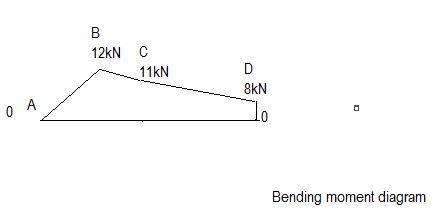Vmax= -10kN (I can see this from the shear force diagram)
Mmax = -11kN.m (point c?)
Points of contra-flexure = C (not sure how this is calculated)

In your shear force diagram, why does the line slope down from -6 to -10? Wouldn't that be for a uniformly applied load of 4kN along AB?
SF @ D = 12kN (same as C)
How do you get that?
BM@B=6kNx1+12kNx0.5 = 12kN CCW
This is not how to calculate bending moments. You should only consider forces on one side of the point. Which side does not matter in principle as long as you are consistent. Switching sides will just flip the sign.

•DevonZA
In your shear force diagram, why does the line slope down from -6 to -10? Wouldn't that be for a uniformly applied load of 4kN along AB?

How do you get that?

This is not how to calculate bending moments. You should only consider forces on one side of the point. Which side does not matter in principle as long as you are consistent. Switching sides will just flip the sign.

Is the 4kN not added to the 6kN therefore giving us 10kN?

The shear force at D I thought would be the same as at C because there are no further forces between C and D?

Looking at the RHS for bending moments:
BM@A=-4kNx1+12kNx1.5=14kN CCW
BM@B=612kNx0.5 = 6kN CCW
BM@C=0

I am not sure how the bending moment diagram is supposed to look but I would assume something like this;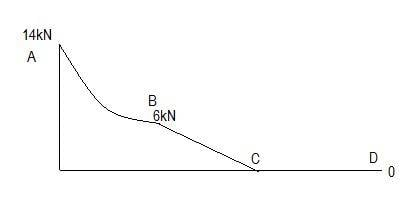Is the 4kN not added to the 6kN therefore giving us 10kN?
Yes, but not until you reach that point in the beam.
For point loads only to the left of some point, the shear diagram up to there should look like a step function and the bending moment would consist of straight line slopes.
With one or more uniform loads to the left, the shear gives straight line slopes while the bending moment has quadratics (parabolas).
Looking at the RHS for bending moments:
No, don't do that - stick with working from the left. That seems to be standard and so you may lose marks doing something different.

•DevonZA
Yes, but not until you reach that point in the beam.
For point loads only to the left of some point, the shear diagram up to there should look like a step function and the bending moment would consist of straight line slopes.
With one or more uniform loads to the left, the shear gives straight line slopes while the bending moment has quadratics (parabolas).

No, don't do that - stick with working from the left. That seems to be standard and so you may lose marks doing something different.

Like this: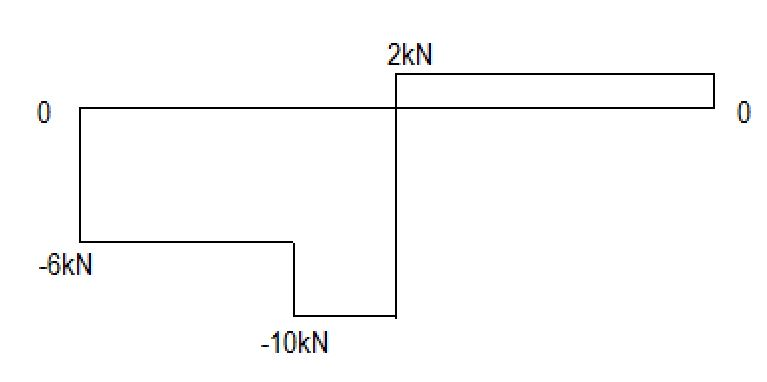LHS

BM@A=0
BM@B=6kNx1=6kN CCW
BM@C=6kNx1.5+4kNx0.5=11kN CCW
BM@D=6kNx3+4kNx2-12kNx1.5=8kN CCW

I really don't know what the bending moment diagram should look like though?

Like this:

View attachment 139475

LHS

BM@A=0
BM@B=6kNx1=6kN CCW
BM@C=6kNx1.5+4kNx0.5=11kN CCW
BM@D=6kNx3+4kNx2-12kNx1.5=8kN CCW

I really don't know what the bending moment diagram should look like though?
You shear diagram and your moments for the individual points are correct.
The bending moment diagram should be continuous - no steps.
To do it properly you should consider a point at between A and B, at distance x from A say, and calculate the bending moment there. Then do likewise for a general point between B and C, etc.
But knowing that for point loads it is all straight lines, you can cheat and just connect up the plotted individual points.

•DevonZA
You shear diagram and your moments for the individual points are correct.
The bending moment diagram should be continuous - no steps.
To do it properly you should consider a point at between A and B, at distance x from A say, and calculate the bending moment there. Then do likewise for a general point between B and C, etc.
But knowing that for point loads it is all straight lines, you can cheat and just connect up the plotted individual points.

Something like this: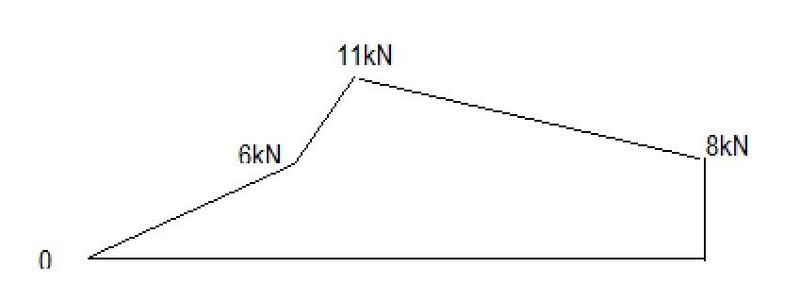Thanks again for your help Haruspex